Journal of Vibroengineering

Published: 11 August 2021

# Optimization of trajectory tracking control of 3-DOF translational robot use PSO method based on inverse dynamics control for surgery application

Ngoc Pham Van Bach1
Quan Dam Hai2
Thanh Bui Trung3
1, 2Space Technology Institute, Hanoi, Vietnam
3Faculty of Mechanical engineering, Hung Yen University of Technology and Education, Hung Yen, Vietnam
Corresponding Author:
Ngoc Pham Van Bach
Views 275

#### Abstract

This research presents an optimal trajectory tracking control method for improving the accuracy of 3-DOF translational parallel robots in the surgery field based on the Particle Swarm Optimization (PSO) method. The 3-DOF translational robot has three translational degrees of freedom, which consists of three arms with three revolute joints and twelve spherical joints. Firstly, the kinematics model is established; and the dynamics equation of the Robot is built by applying the Lagrange equation of the first type, and then the dynamics controller of 3-DOF translational robot is designed base on the dynamics equations. Secondly, a trajectory tracking controller model using the Particle Swarm Optimization based on inverse dynamics controlled method for 3-DOF translational Robot is designed. The control performance results of the proposed controller is evaluated by simulation and compared with the other published research results. Finally, the proposed controller can achieve better tracking performance in comparison with other controllers as Proportional Integral Derivative (PID) controller, GPI controller, and adaptive controller.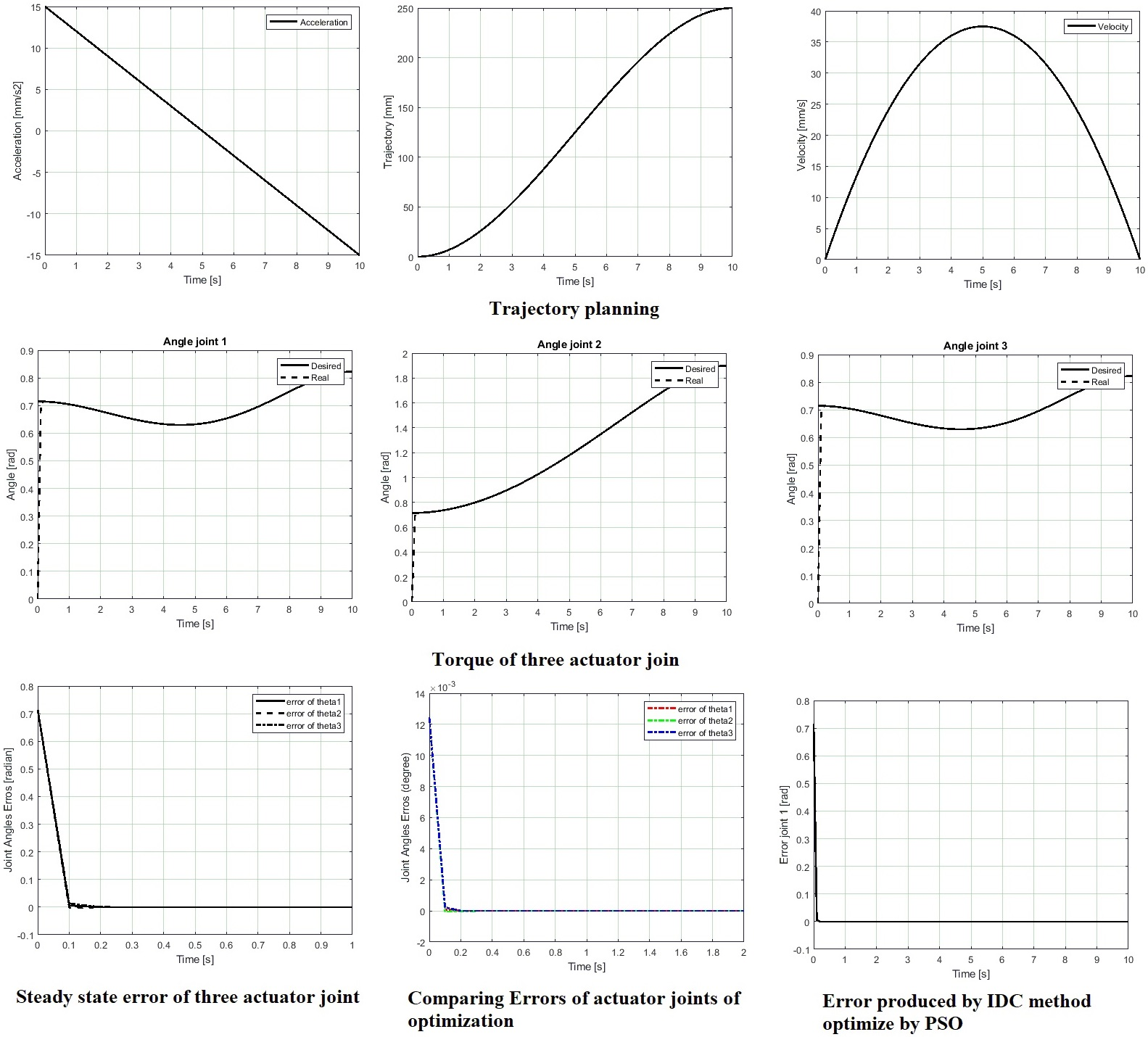#### Highlights

• A trajectory tracking controller model using the Particle Swarm Optimization based on inverse dynamics controlled method for 3-DOF translational Robot
• Proportional Integral Derivative (PID) controller, GPI controller, and adaptive controllers optimization method, Integral of the Square of the Error (ISE) play an important role as objective conditions
• The IDC is a novel and effective method for 3-DOF translational robot control systems in the surgery field trajectory tracking of 3-DOF translational robot problems was implemented and optimized by used the Inverse Dynamics Control (IDC) method and Particle Swarm Optimization (PSO) algorithm
• PSO algorithm to find out the gains of controller more quick and simple than other methods

## 1. Introduction

This paper organizes like this: presents an optimal trajectory tracking control method for 3-DOF translational robot Delta type. The 3-DOF translational robot with three translational degrees of freedom has chosen. First of all, mechanism, kinematic and dynamic of robot are presented. Secondly, based on the dynamic of 3-DOF translational robot, an inverse dynamic controller (IDC) is designed to track a desired trajectory. To achieve the best performance of trajectory control, the Particle Swarm Optimization (PSO) is used to determine parameters of the controller. In this optimization method, Integral of the Square of the Error (ISE) play an important role as objective conditions. The simulation results are presented and compared with other control methods in the rest of this article.

## 2. Mathematic of kinematics and dynamics of 3-DOF translational robot

### 2.1. Kinematic of 3-DOF Translational robot

The 3-DOF translational robot has three arm connect the moving platform and the fixed base is shown in Fig. 1. Each arm have two parts, the upper arm is connected to the active motor using revolute joint. Each lower arm is made up of a four-bar parallelogram which connect with the moving platform and the upper arm using spherical joint. The moving platform can perform translational movement in three directions. In other words, the robot is called three translational degrees of freedom.

Fig. 13D drawing of 3-DOF translational robot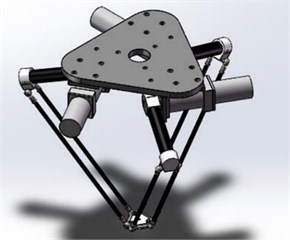Fig. 2Vectors description of 3-DOF translational robot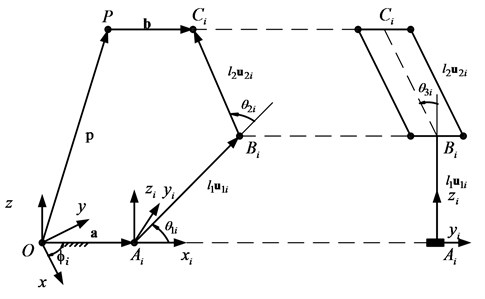Kinematics of 3-DOF translational robot has discussed by many authors with many methods, in this study authors chose the vector loop equation method to solve this problem. In , authors proposed the inverse kinematics of Delta parallel robot base on vectors loop equation. Vectors of 3-DOF translational robot are illustrated in Fig. 2. As shown on Fig. 2, the vectors loop equation of parallel robot is given as follow:

1
${l}_{1i}{u}_{1i}+{l}_{2i}{u}_{2i}=p+b-a.$

Expressing Eq. (1) in the $\left({x}_{i},{y}_{i},{z}_{i}\right)$ frame with $i=1,2,3$ yield:

2
$\left[\begin{array}{c}{l}_{1}\mathrm{c}\mathrm{o}\mathrm{s}{\theta }_{1i}+{l}_{2}\mathrm{s}\mathrm{i}\mathrm{n}{\theta }_{3i}\mathrm{c}\mathrm{o}\mathrm{s}\left({\theta }_{1i}+{\theta }_{2i}\right)\\ {l}_{2}\mathrm{c}\mathrm{o}\mathrm{s}{\theta }_{3i}\\ {l}_{1}\mathrm{s}\mathrm{i}\mathrm{n}{\theta }_{1i}+{l}_{2}\mathrm{s}\mathrm{i}\mathrm{n}{\theta }_{3i}\mathrm{s}\mathrm{i}\mathrm{n}\left({\theta }_{1i}+{\theta }_{2i}\right)\end{array}\right]=\left[\begin{array}{l}{c}_{xi}\\ {c}_{yi}\\ {c}_{zi}\end{array}\right],$

where ${l}_{1},{l}_{2}$ are length of lower arm and upper arm; ${\theta }_{1i},{\theta }_{2i},{\theta }_{3i}$ with $\left(i=1,2,3\right)$ are actuated joint angles and passive joint angles respectively, ${c}_{xi},{c}_{yi},{c}_{zi}$ are position of point ${C}_{i}$. The position of ${C}_{i}$ can be obtained as:

3
$\left[\begin{array}{l}{c}_{xi}\\ {c}_{yi}\\ {c}_{zi}\end{array}\right]=\left[\begin{array}{ccc}\mathrm{c}\mathrm{o}\mathrm{s}{\phi }_{i}& \mathrm{s}\mathrm{i}\mathrm{n}{\phi }_{i}& 0\\ -\mathrm{s}\mathrm{i}\mathrm{n}{\phi }_{i}& \mathrm{c}\mathrm{o}\mathrm{s}{\phi }_{i}& 0\\ 0& 0& 1\end{array}\right]\left[\begin{array}{l}{p}_{x}\\ {p}_{y}\\ {p}_{z}\end{array}\right]+\left[\begin{array}{c}a-b\\ 0\\ 0\end{array}\right],$

where ${p}_{x},{p}_{y},{p}_{z}$ are position of the center point of moving platform in the $\left(x,y,z\right)$coordinate system. The solutions of ${\theta }_{1i},{\theta }_{2i},{\theta }_{3i}$ are found by solving Eq. (2).

Solving Eq. (2) obtained:

4
${\theta }_{3i}=\mathrm{a}\mathrm{r}\mathrm{c}\mathrm{c}\mathrm{o}\mathrm{s}\left(\frac{{c}_{yi}}{{l}_{2}}\right),$
5
${\theta }_{2i}=\mathrm{a}\mathrm{r}\mathrm{c}\mathrm{c}\mathrm{o}\mathrm{s}\left(\kappa \right),$

where $\kappa =\left({c}_{xi}^{2}+{c}_{yi}^{2}+{c}_{zi}^{2}-{l}_{1}^{2}-{l}_{2}^{2}\right)/\left(2{l}_{1}{l}_{2}\mathrm{s}\mathrm{i}\mathrm{n}{\theta }_{3i}\right)$.

Substituting Eq. (4) and Eq. (5) into Eq. (2):

6
${l}_{1}\mathrm{s}\mathrm{i}\mathrm{n}{\theta }_{1i}+{l}_{2}\mathrm{s}\mathrm{i}\mathrm{n}{\theta }_{3i}\mathrm{s}\mathrm{i}\mathrm{n}\left({\theta }_{1i}+{\theta }_{2i}\right)={c}_{zi},$
7
${l}_{1}\mathrm{c}\mathrm{o}\mathrm{s}{\theta }_{1i}+{l}_{2}\mathrm{s}\mathrm{i}\mathrm{n}{\theta }_{3i}\mathrm{c}\mathrm{o}\mathrm{s}\left({\theta }_{1i}+{\theta }_{2i}\right)={c}_{xi}.$

Solving two equations above yield:

8
${\theta }_{1i}=\mathrm{a}\mathrm{r}\mathrm{c}\mathrm{t}\mathrm{a}\mathrm{n}\frac{-{c}_{xi}{g}_{2i}+{c}_{zi}{g}_{1i}}{{c}_{zi}{g}_{2i}+{c}_{xi}{g}_{1i}},$

where ${g}_{2i}={l}_{2}\mathrm{s}\mathrm{i}\mathrm{n}{\theta }_{3i}\mathrm{s}\mathrm{i}\mathrm{n}{\theta }_{2i}$, ${g}_{1i}=\left({l}_{1}+{l}_{2}\mathrm{s}\mathrm{i}\mathrm{n}{\theta }_{3i}\mathrm{c}\mathrm{o}\mathrm{s}{\theta }_{2i}\right)$.

### 2.2. Dynamics equation of 3-DOF translational robot

In this research author using the Lagrangian formulation of the first type to solve the dynamics problems of 3-DOF translational robot. The Lagrangian function of the first type is written as :

9

where ${\mathrm{\Gamma }}_{i}$denotes the $i$th constraint function, $k$ is the number of constraint functions, ${\lambda }_{i}$ is the Lagrangian multiplier. $L$ is the Lagrangian function. Constraint equations ${\mathrm{\Gamma }}_{i}$ with $i=1,2,3$ are obtained from the fact that the distance between joints B and C is always equal to the length of the connecting rod of the upper arm:

10

Taking the derivatives of contraint functions and Lagrangian function, Lagrangian multipliers, the dynamics of 3-DOF translational robot can be written as:

11
${\tau }_{1}=\left({I}_{m}+{I}_{1}+{m}_{2}{l}_{1}^{2}\right){\stackrel{¨}{\theta }}_{11}+\left({m}_{1}{l}_{1c}+{m}_{2}{l}_{1}\right){g}_{c}\mathrm{c}\mathrm{o}\mathrm{s}{\theta }_{11}$

where ${I}_{m}$ is axial moment of inertia of the rotor mounted on the $i$th limb, ${I}_{1}$is moment of lower arms, ${m}_{p}$, ${m}_{1}$, ${m}_{2}$ are mass of moving platform, lower arm and upper arm respectively Eq. (11) can be rewritten in matrix form as following:

12
$M\left(q\right)\stackrel{¨}{q}+G\left(q\right)=\tau ,$

where: $M$ is the manipulator inertia matrix, $G\left(q\right)$ is the gravitational vector, $\tau$ is the vector of generalized forces, $q$ is vector of generalized Lagrange coordinates:

## 3. Optimization trajectory tracking controller of 3-DOF translational robot

### 3.1. Controller design

The dynamics Eq. (11) can be rewritten in matrices form as below:

13
$M\left(q\right)\stackrel{¨}{q}+C\left(q,\stackrel{˙}{q}\right)\stackrel{˙}{q}+G\left(q\right)=\tau +{\tau }_{d},$

where $M\left(q\right)$,$C\left(q,\stackrel{˙}{q}\right)$, $G\left(q\right)$ are mass matrix, Coriolis and Centrifugal matrix, gravity vector respectively, ${\tau }_{d}$ is disturbance.

The Inverse Dynamics control law of 3-DOF transnational robot is written as :

14
$\tau =\stackrel{^}{M}\left(q\right)\left({\stackrel{¨}{q}}_{d}+{K}_{p}e+{K}_{d}\stackrel{˙}{e}\right)+\stackrel{^}{C}\left(q,\stackrel{˙}{q}\right)\stackrel{˙}{q}+\stackrel{^}{G}\left(q\right),$

where $\stackrel{^}{M}\left(q\right),\stackrel{^}{C}\left(q,\stackrel{˙}{q}\right),\stackrel{^}{G}\left(q\right)$ denote the approximate estimate of the manipulator mass matrix, Coriolis and Centrifugal matrix and gravity vector in the joint space respectively; ${q}_{d}$ and ${\stackrel{¨}{q}}_{d}$ are joint angle and joint acceleration desired; ${K}_{d}$, ${K}_{p}$ are positive diagonal matrices.

Substituting Eq. (9) into Eq. (8) the dynamics equation of 3-DOF translational robot can be expressed as follow:

15
$M\left(q\right)\stackrel{¨}{q}+C\left(q,\stackrel{˙}{q}\right)\stackrel{˙}{q}+G\left(q\right)=\tau +{\tau }_{d}$

In the dynamics equation of 3-DOF translational robot $C\left(q,\stackrel{˙}{q}\right)=0$ thus $\stackrel{^}{C}\left(q,\stackrel{˙}{q}\right)=0$. In real model, the $M\left(q\right)$ and $G\left(q\right)$ can calculated, assume that $\stackrel{^}{M}\left(q\right)=M\left(q\right)$, $\stackrel{^}{G}\left(q\right)=G\left(q\right)$ and there is no disturbance wrench is applied to the manipulator, the error dynamic is simplified to:

16
${\stackrel{¨}{q}}_{d}+{K}_{d}\stackrel{˙}{e}+{K}_{p}e=0.$

With error dynamic equation Eq. (16) above, the tracking error can be designed to convergence toward zero by choosing ${K}_{d}$, ${K}_{p}$ appropriate. The Inverse Dynamics controller model in joint space is depicted in Fig. 3.

This section may be divided by subheadings. It should provide a concise and precise description of the experimental results, their interpretation, as well as the experimental conclusions that can be drawn.

Fig. 3Trajectory tracking inverse dynamics controller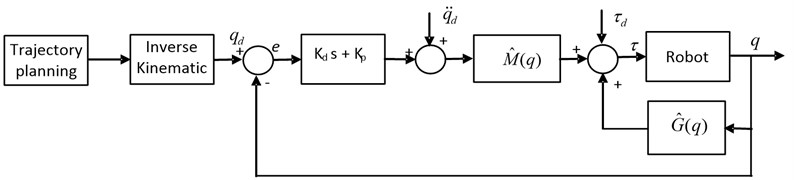### 3.2. Particle Swarm Optimization (PSO) algorithm

This paper is presented a method of choosing the parameters of the Inverse Dynamics Controller. The dynamics error equation reach to zero by choosing ${K}_{d}$, ${K}_{p}$ gains. In this research, the authors are using the Particle Swarm Optimization method to find the optimization gains. The advantages of PSO are: simplicity and efficiency, proven in many other parameters training problems. The PSO method was developed by simulation of simplified social model, where each population is called a swarm. Each candidate, called a particle, flies through problem space to look for the optimal position, similar to food searching of bird swarm. Assume that, the search space is n-dimension, each particle $i$ corresponding to an $n$-dimension position vector ${x}_{i}=\left(\begin{array}{llll}{x}_{i1},& {x}_{i2},& ...,& {x}_{in}\end{array}\right)$, and velocity is represented by an $n$-dimension velocity vector, ${v}_{i}=\left(\begin{array}{llll}{v}_{i1},& {v}_{i2},& ...,& {v}_{in}\end{array}\right)$. The best previously visited position $i$ is called individual best position, ${P}_{i}=\left(\begin{array}{llll}{p}_{i1},& {p}_{i2},& ...,& {p}_{in}\end{array}\right)$. The position of the best individual of the whole swarm is called the global best position, $G=\left(\begin{array}{llll}{g}_{1},& {g}_{2},& ...,& {g}_{n}\end{array}\right)$. The velocity and position are updated at each step according to the following two equations:

17
18
${x}_{i}\left(k+1\right)={x}_{i}\left(k\right)+{v}_{i}\left(k\right),$

where $w$ is inertia weight; ${r}_{1}$, ${r}_{2}$ are random variables in the range of $\left[\begin{array}{ll}0& 1\end{array}\right]$; ${c}_{1}$, ${c}_{2}$ are positive constant parameters called acceleration coefficients.

In this paper, the PSO was combined with the kinematic and dynamic model of 3-DOF translational robot to find out the gains of controller. The parameters was chosen as $w=\text{0.9}$, ${c}_{1}=\text{0.12}$, ${c}_{2}=\text{1.2}$, $n=\text{50}$, $k=\text{50}$. The fitness function is the measurement of the trajectory’s quality. The objective functions applied for this particular problem is the Integral Square Error (ISE). In this case, the best position is determined by the minimum of Integral of the Square of the Error value by using Matlab toolbox. In each iteration, the gains are updated sequentially in individual best position set and global best position set corresponding to ISE value. The gains are taken after 50 iterations show the errors are acceptable as mention in simulation part below.

## 4. Simulation results and discussion

The simulation of kinematic, dynamic and control of the 3-DOF translational robot was implemented in Matlab/Simulink. Dynamic parameters of the robot are described in Table 1.

As mention above, corresponding to dynamic parameters of the robot, the ${K}_{d}$, ${K}_{p}$of the controller was found by applying the PSO method, we have:

Table 1Parameter of 3-DOF translational

 Parameter Value Unit Mass of lower arm ${m}_{1}$ 0.426 kg Mass of upper arm ${m}_{2}$ 0.069 kg Mass of moving platform ${m}_{p}$ 0.069 kg Length of lower arm ${l}_{1}$ 171.5 mm Length of upper arm ${l}_{2}$ 396 mm Radius of fix platform $a$ 100 mm Radius of moving platform $b$ 22.5 mm

The trajectory form was designed by using the third odder polynomial as below :

19
$q\left(t\right)={a}_{0}+{a}_{1}t+{a}_{2}{t}^{2}+{a}_{3}{t}^{3}.$

The coefficients of Eq. (14) are obtained by solving set of equations Eq. (15):

20
$\left[\begin{array}{llll}1& {t}_{0}& {t}_{0}^{2}& {t}_{0}^{3}\\ 0& 1& 2{t}_{0}& 3{t}_{0}^{2}\\ 1& {t}_{f}& {t}_{f}^{2}& {t}_{f}^{3}\\ 0& 1& 2{t}_{f}& 3{t}_{f}^{2}\end{array}\right]\left[\begin{array}{l}{a}_{0}\\ {a}_{1}\\ {a}_{2}\\ {a}_{3}\end{array}\right]=\left[\begin{array}{l}{q}_{0}\\ {v}_{0}\\ {q}_{f}\\ {v}_{f}\end{array}\right],$

where $q\left({t}_{0}\right)={q}_{0}$, $\stackrel{˙}{q}\left({t}_{0}\right)={v}_{0}$, $q\left({t}_{f}\right)={q}_{f}$, $\stackrel{˙}{q}\left({t}_{f}\right)={v}_{f}$ and ${t}_{0}$, ${q}_{0}$,${v}_{0}$ are initial time, position and velocity respectively, ${t}_{f}$, ${q}_{f}$,${v}_{f}$ are final time, position and velocity respectively.

In this research, the trajectory of the moving platform is implemented in $OX$ direction which relative to the $\left(x,y,z\right)$ coordinate system, where ${t}_{0}=0$, ${t}_{f}=$ 10 (sec), ${q}_{0}=0$, ${q}_{f}=$250 (mm) and ${v}_{0}=0$, ${v}_{f}=0$. The trajectory, velocity and acceleration are showed in Fig. 4.

Fig. 4Trajectory planning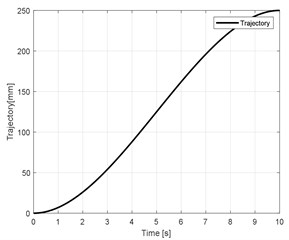a)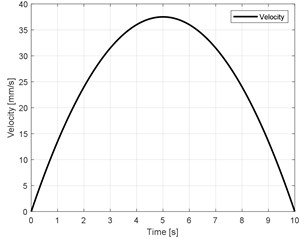b)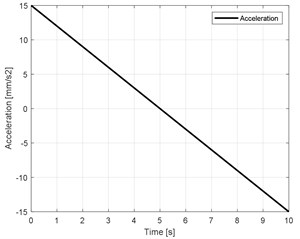c)

The numerical simulation results of the joint angles of three arm are shown in Figs. 5(a-c). The torque of three actuators joint are presented in Fig. (d).

Fig. 5Torque of three actuator joint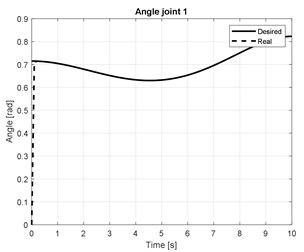a)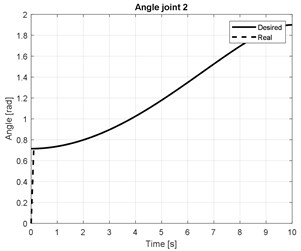b)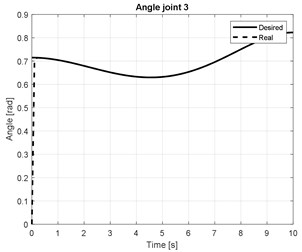c)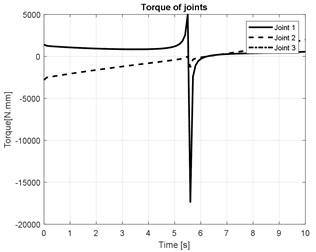d)

As mention at the first part, the objective condition of optimization method is integral of the square of the error ${J}_{ISE}$. The PSO will apply to find ${K}_{d}$, ${K}_{p}$which corresponds to minimum of ${J}_{ISE}$ :

21
${J}_{ISE}=\underset{0}{\overset{+\infty }{\int }}{e}_{ss}^{2}dt,$

where ${e}_{ss}$ is steady state error of actuator joint of the robot. According to parameters and the trajectory planning of the Delta robot, the steady state error of three actuators joints are showed in Fig. 6. Where ${J}_{ISE1}=$ 0.2555, ${J}_{ISE3}=$ 0.2557 respectively.

Fig. 6Steady state error of three actuator joint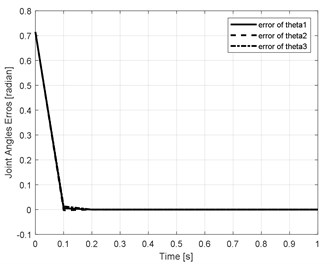The performance of proposed control method could be evaluated by compare with other methods in  as shown below in Fig. 7.

Fig. 7Comparing angle error with other methods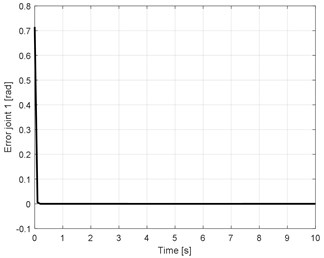a) Error produced by IDC method optimize by PSO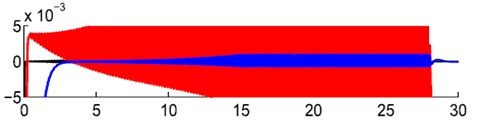b) Red line is error produced by PID controller, blue line is error produced by GPI controller (base on extended state observer), black line is error produced by adaptive controller proposed in 

Fig. 7 shows the error of actuator joint 1 of the proposed controller and other controllers implemented in . Fig. 7(b) shows that all results still oscillate around zero points with 0.005 rad deviation. The result of adaptive controller is the best of three methods but still restricted to a vicinity of the origin. Fig. 7(a) shows the error produced by optimization IDC is better than other methods. The graphs show that the error converges to zero after 0.2 s and the steady-state error is maintained no more than 5.10-5 rad. Another comparison can be performed between the proposed controller and the controller in , the graph of errors as showed in Fig. 8.

Fig. 8Comparing Errors of actuator joints of optimization a) IDC and b) PD-CTC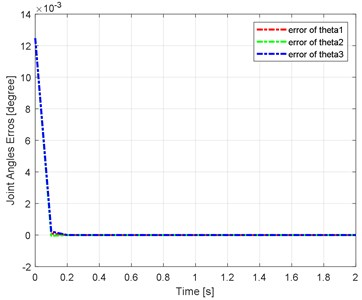a)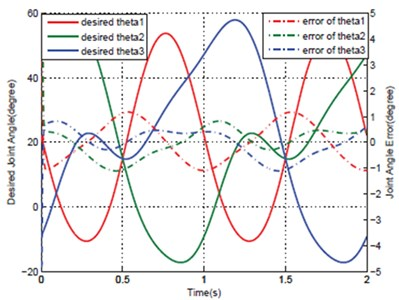b) The maximum error of joints at 1 degree (0.017 rad) while in a) as mention above, the error is 5.10-5 rad

Fig. 8 show the error of three actuators joint angle of the 3-DOF translational robot. In Fig. 8(a), the error curve converges to zero after 0.1sec, and the steady state error is very small, while in Fig. 8(b), this value cannot converge to zero, even it oscillates around –1 and 1 degree. The comparisons above demonstrates the better performance of this controller in accuracy and stability.

## 5. Conclusions

In this paper, the trajectory tracking of 3-DOF translational robot problems was implemented and optimized by used the Inverse Dynamics Control (IDC) method and Particle Swarm Optimization (PSO) algorithm. The IDC is used to track the desired trajectory in joint space. The IDC is a novel and effective method for 3-DOF translational robot control systems in the surgery field. PSO algorithm to find out the gains of controller more quick and simple than other methods. The comparison of simulation results showed the steady-state error of the proposed method is smaller and converge to zero faster than other methods. In the future, this control method will be used to combine with other force control to improve the control of the 3-DOF translational robot in the real model.

#### Acknowledgements

This work is funded by VAST project under grant number VAST01.06/19-20.